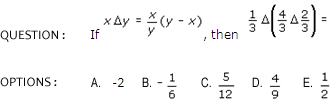# KI Unique Quantitative SectionGRE Exam
Explore more-GRE
KI Unique
Test Yourself
Help

## KI Unique Quantitative Section

Quantitative Section

The quantitative section basically covers:

Arithmetic, Algebra & Basic Geometry
• The level of mathematics is not more advanced then high school mathematics but it involves extra reasoning skills and knowledge.
• Time Management is very imp
• erative. Easier questions should be allotted less than two minutes and difficult ones should be then given more time, accordingly.
1. Problem Solving
1. If the question asked for a numerical value, the answer choices will increase in size as you read down the list. So if you estimate roughly the size of number the question asks for, then you can easily zero in on the most viable answer choices.
2. All the short cut formulas related to arithmetic, algebra etc. should be on tips.
3. Practice the questions keeping a time format in mind while preparing for Problem solving so that you can complete your exam at time.
4. Don't get carried away with detailed calculations. Look for a trick or a shortcut if the question seems time consuming.
5. Example of Conventional Problem solving Format Quest2. Quantitative Comparisons
1. Factor out, and then cancel, any common expressions or quantities in both Columns A and B. Remember that you are just trying to make relative comparisons.
2. Compare piece by piece.
3. Make one column look like other
4. Pick numbers.
5. Redraw diagrams.

 COLUMN A COLUMN B x(x-1) (x2-x)

Ans: Both columns are equal. Column B is the result of Column A.

Copy Right © 2012 by Knowledge Icon
Website Designed & Developed By Dream Business Systems